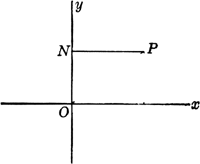# abscissa

Also found in: Dictionary, Thesaurus, Medical, Wikipedia.
Related to abscissa: Abscissa of convergence

## Cartesian coordinates

Cartesian coordinates (kärtēˈzhən) [for René Descartes], system for representing the relative positions of points in a plane or in space. In a plane, the point P is specified by the pair of numbers (x,y) representing the distances of the point from two intersecting straight lines, referred to as the x-axis and the y-axis. The point of intersection of these axes, which are called the coordinate axes, is known as the origin. In rectangular coordinates, the type most often used, the axes are taken to be perpendicular, with the x-axis horizontal and the y-axis vertical, so that the x-coordinate, or abscissa, of P is measured along the horizontal perpendicular from P to the y-axis (i.e., parallel to the x-axis) and the y-coordinate, or ordinate, is measured along the vertical perpendicular from P to the x-axis (parallel to the y-axis). In oblique coordinates the axes are not perpendicular; the abscissa of P is measured along a parallel to the x-axis, and the ordinate is measured along a parallel to the y-axis, but neither of these parallels is perpendicular to the other coordinate axis as in rectangular coordinates. Similarly, a point in space may be specified by the triple of numbers (x,y,z) representing the distances from three planes determined by three intersecting straight lines not all in the same plane; i.e., the x-coordinate represents the distance from the yz-plane measured along a parallel to the x-axis, the y-coordinate represents the distance from the xz-plane measured along a parallel to the y-axis, and the z-coordinate represents the distance from the xy-plane measured along a parallel to the z-axis (the axes are usually taken to be mutually perpendicular). Analogous systems may be defined for describing points in abstract spaces of four or more dimensions. Many of the curves studied in classical geometry can be described as the set of points (x,y) that satisfy some equation f(x,y)=0. In this way certain questions in geometry can be transformed into questions about numbers and resolved by means of analytic geometry.

## abscissa

[ab′sis·ə]
(mathematics)
One of the coordinates of a two-dimensional coordinate system, usually the horizontal coordinate, denoted by x.
McGraw-Hill Dictionary of Scientific & Technical Terms, 6E, Copyright © 2003 by The McGraw-Hill Companies, Inc.

## abscissaabscissa: P, any point; NP, abscissa
In the plane Cartesian coordinate system, the horizontal coordinate of a point on a plane; the x-coordinate, obtained by measuring the distance from the point to the y-axis along a line parallel to the x-axis.
McGraw-Hill Dictionary of Architecture and Construction. Copyright © 2003 by McGraw-Hill Companies, Inc.

## abscissa

the horizontal or x-coordinate of a point in a two-dimensional system of Cartesian coordinates. It is the distance from the y-axis measured parallel to the x-axis
Collins Discovery Encyclopedia, 1st edition © HarperCollins Publishers 2005

## abscissa

(mathematics)
The horizontal or x coordinate on an (x, y) graph; the input of a function against which the output is plotted.

The vertical or y coordinate is the "ordinate".

See Cartesian coordinates.
References in periodicals archive ?
Caption: Figure 3--Relationship between the Coefficient of Variation (%) for the weight of the marketable cormels and plot size in Basic Units, to determine the abscissa value at the maximum curvature point ([X.sub.o]).
(The abscissa axis shows the frequency in Hz and the ordinate axis shows V/(rad/sec)).
Similarly, in the log-log coordinate system, [p.sub.D] is calculated based on the definition of dimensionless pressure derivative [p'.sub.D]=[dp.sub.D]/d([t.sub.D][e.sup.2S]) A group of pressure derivative type curves (Figure 3) are drawn, with [t.sub.D][e.sup.2S] * [[p'.sub.D].sup.as] as ordinate against [t.sub.D][e.sup.2S] as abscissa and [b.sub.D][e.sup.-2S] as the curve parameter.
Assuming a spectrum band with a unique SPR peak, the FWHM quantity (green line) is given by the abscissas (x-coordinates) of the spectrum equivalent to half-height (HH), which is half the distance between the SPR maximum absorbance and P3.
We set abscissa c = 1, [C.sub.2] = -[10.sup.-5] as the error constant of the method and use relations (2.3) and (2.5) for satisfying order condition.
In the paper "Stability and Multiscroll Attractors of Control Systems via the Abscissa," E.-C.
The pitch angle will particularly exist throughout [-[pi], [pi]] once the abscissa [x.sup.+.sub.10] > 7113 m.
where Id is the edge identifier in the graph database, Dp is the curvilinear abscissa, [delta] represents the travel direction, which can be 1 if the edge is walked forward (from A to B) and -1 otherwise (from B to A), k is the scale factor that calibrates the step length model, [beta] is the misalignment between the walking direction and the pointing direction of the handheld device, w is the particle weight, and p and t refer, respectively, to the particle and time.
Taking the liquid volume flow rate and PE_H as the abscissa and the ordinate respectively, the piecewise linear interpolation model is established and denoted as PH model.
Using the pH as the abscissa and the OD value of bacterial suspension as the ordinate, we obtained the growth curves of the test bacteria at various pH values (10).

Site: Follow: Share:
Open / Close